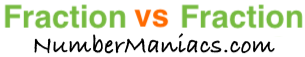3/4 vs 1/4People compare 3/4 vs 1/4 drywall, 3/4 vs 1/4 pipe, 3/4 vs 1/4 cup, 3/4 vs 1/4 ton, 3/4 vs 1/4 drill bit, 3/4 vs 1/4 poker, 3/4 vs 1/4 sump pump, 3/4 vs 1/4 garden hose, and many other items.

Is 3/4 greater than 1/4? Is 3/4 less than 1/4? What is the difference between 3/4 and 1/4? These and other questions are answered below so you can get a better perspective of 3/4 vs 1/4.

3/4 vs 1/4 Fractional Comparisons
3/4 is more than 1/4
The difference between 3/4 and 1/4 is 1/2
3/4 is 1/2 more than 1/4

3/4 vs 1/4 Decimal Comparisons
3/4 vs 1/4 as a decimal is 0.75 vs 0.25
The difference between 3/4 and 1/4 is 0.5
3/4 is 0.5 more than 1/4

3/4 vs 1/4 Percent Comparisons
3/4 vs 1/4 as a percent is 75% vs 25%
3/4 is 200 percent more than 1/4

Fraction vs Fraction
Submit another set of fractions that we can compare for you!

/
vs
/

The math behind the numbers
Calculations on this page are rounded to the nearest thousandth if necessary. Below is the math we used to analyze 3/4 vs 1/4:

To compare the size of 3/4 vs 1/4, we simply subtracted 1/4 from 3/4:

3/4 - 1/4 = |1/2|

To find 3/4 vs 1/4 as a decimal, we divided the numerator by the denominator for each fraction.

3 ÷ 4 = 0.75
1 ÷ 4 = 0.25

To find 3/4 vs 1/4 as a percent, we divided the numerator by the denominator and then multiplied the quotient by 100 for each fraction.

(3 ÷ 4) x 100 = 75%
(1 ÷ 4) x 100 = 25%

We found that 3/4 is 200 percent more than 1/4 by dividing the difference between the two fractions by the second fraction and then multiplied it by 100:

((1/2)/(1/4))×100 = 200%

That's it folks. We hope this page gave you a better understanding of 3/4 vs 1/4 with our explanations and conclusions.

3/4 vs 1/5
Here are the next two fractions we have compared and analyzed.